Next: Optical Theorem Up: Scattering Theory Previous: Born Expansion

# Partial Waves

We can assume, without loss of generality, that the incident wavefunction is characterized by a wavevector,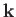, that is aligned parallel to the-axis. The scattered wavefunction is characterized by a wavevector,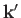, that has the same magnitude as, but, in general, points in a different direction. The direction ofis specified by the polar angle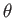(i.e., the angle subtended between the two wavevectors), and an azimuthal angle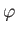measured about the-axis. Equations (10.33) and (10.34) strongly suggest that for a spherically symmetric scattering potential [i.e.,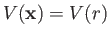], the scattering amplitude is a function ofonly: that is,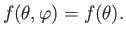(10.53)

Let us assume that this is the case. It follows that neither the incident wavefunction,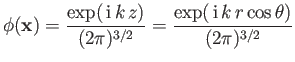(10.54)

[see Equation (10.14)], nor the total wavefunction far from the scattering region,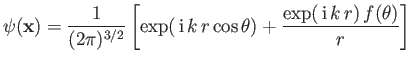(10.55)

[see Equation (10.20)], depend on the azimuthal angle,.

Outside the range of the scattering potential,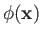and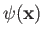both satisfy the free-space Schrödinger equation,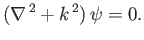(10.56)

Consider the most general solution to this equation that is independent of the azimuthal angle,. Separation of variables (in spherical coordinates) yields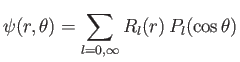(10.57)

(See Exercise 10.) The Legendre polynomials,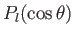, are related to the associated Legendre functions,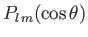, as well as the spherical harmonics,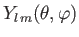, introduced in Section 4.4, via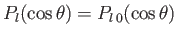, and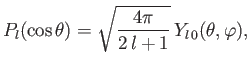(10.58)

respectively. Equations (10.56) and (10.57) can be combined to give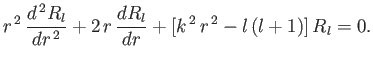(10.59)

(See Exercise 10.) The two independent solutions to this equation are the spherical Bessel function,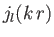, and the Neumann function,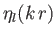, where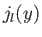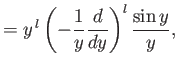(10.60)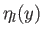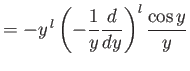(10.61)

. Note that spherical Bessel functions are well behaved in the limit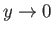, whereas Neumann functions become singular. The asymptotic behavior of these functions in the limit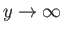is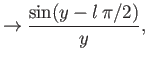(10.62)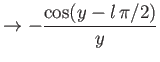(10.63)

.

We can write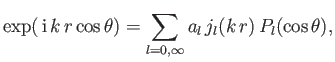(10.64)

where the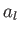are constants. Of course, there are no Neumann functions in this expansion because they are not well behaved as(whereas the function on the left-hand side is clearly finite at). As is well known, the Legendre polynomials are orthogonal functions,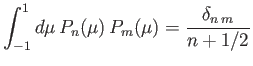(10.65)

, so we can invert the preceding expansion to give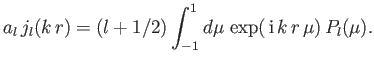(10.66)

Now,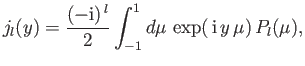(10.67)

for. Thus, a comparison of the previous two equations yields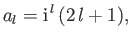(10.68)

giving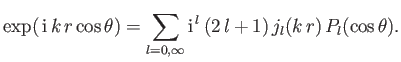(10.69)

The preceding expression specifies how a plane wave can be decomposed into a series of spherical waves. The latter waves are usually referred to as partial waves.

The most general expression for the total wavefunction outside the scattering region is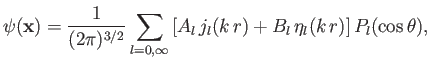(10.70)

where the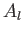and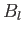are constants. Note that the Neumann functions are allowed to appear in this expansion, because its region of validity does not include the origin. In the large-limit, the total wavefunction reduces to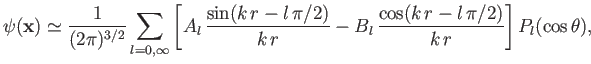(10.71)

where use has been made of Equations (10.62) and (10.63). The previous expression can also be written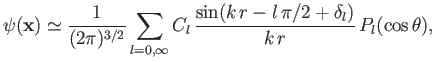(10.72)

where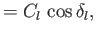(10.73)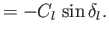(10.74)

Equation (10.72) yields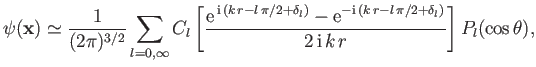(10.75)

which contains both incoming and outgoing spherical waves. What is the source of the incoming waves? Obviously, they must form part of the large-asymptotic expansion of the incident wavefunction. In fact, it is easily seen from Equations (10.54), (10.62), and (10.69) that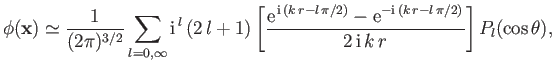(10.76)

in the large-limit. Now, Equations (10.54) and (10.55) give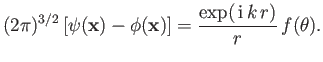(10.77)

Note that the right-hand side consists only of an outgoing spherical wave. This implies that the coefficients of the incoming spherical waves in the large-expansions ofandmust be equal. It follows from Equations (10.75) and (10.76) that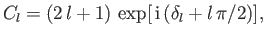(10.78)

which leads to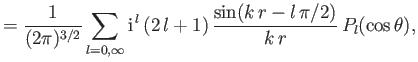(10.79)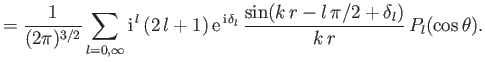(10.80)

Thus, it is apparent that the effect of the scattering is to introduce a phase-shift,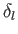, into theth partial wave. Finally, Equation (10.77) yields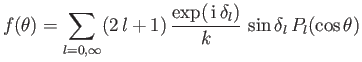(10.81)

. Clearly, determining the scattering amplitude,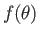, via a decomposition into partial waves (i.e., spherical waves), is equivalent to determining the phase-shifts,.

It is helpful to write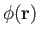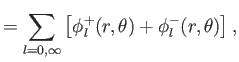(10.82)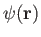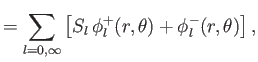(10.83)

where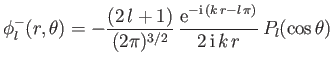(10.84)

is an ingoing spherical wave, whereas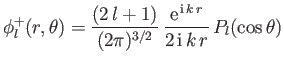(10.85)

is an outgoing spherical wave. Moreover,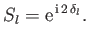(10.86)

[See Equations (10.79) and (10.80).] Note that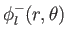and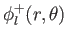are both eigenstates of the magnitude of the total orbital angular momentum about the origin belonging to the eigenvalues. (See Chapter 4.) Thus, in preforming a partial wave expansion, we have effectively separated the incoming and outgoing particles into streams possessing definite angular momenta about the origin. Moreover, the effect of the scattering is to introduce an angular-momentum-dependent phase-shift into the outgoing particle streams.

The net outward particle flux through a sphere of radius, centered on the origin, is proportional to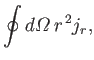(10.87)

where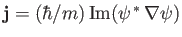is the probability current. It follows that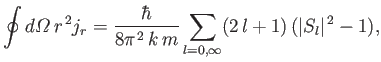(10.88)

where use has been made of Equation (10.65). Of course, the net particle flux must be zero, otherwise the number of particles would not be conserved. Particle conservation is ensured by the fact that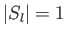for all. [See Equation (10.86).]Next: Optical Theorem Up: Scattering Theory Previous: Born Expansion
Richard Fitzpatrick 2016-01-22